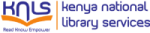Normal view

# Chemical engineering computation with MATLAB / Yeong-Koo Yeo.

Material type:TextPublisher: Boca Raton : CRC Press, 2020Edition: Second editionDescription: 1 online resource : illustrations (black and white)Content type:
• text
• still image
Media type:
• computer
Carrier type:
• online resource
ISBN:
• 9781000284928
• 1000284921
• 9781000284881
• 1000284883
• 9781000284904
• 1000284905
• 9781003090601
• 1003090605
DDC classification:
• 660.0285 23
LOC classification:
• TP184
Online resources: Summary: Chemical Engineering Computation with MATLAB, Second Edition continues to present basic to advanced levels of problem-solving techniques using MATLAB as the computation environment. The Second Edition provides even more examples and problems extracted from core chemical engineering subject areas and all code is updated to MATLAB version 2020. It also includes a new chapter on computational intelligence and: Offers exercises and extensive problem-solving instruction and solutions for various problems Features solutions developed using fundamental principles to construct mathematical models and an equation-oriented approach to generate numerical results Delivers a wealth of examples to demonstrate the implementation of various problem-solving approaches and methodologies for problem formulation, problem solving, analysis, and presentation, as well as visualization and documentation of results Includes an appendix offering an introduction to MATLAB for readers unfamiliar with the program, which will allow them to write their own MATLAB programs and follow the examples in the book Provides aid with advanced problems that are often encountered in graduate research and industrial operations, such as nonlinear regression, parameter estimation in differential systems, two-point boundary value problems and partial differential equations and optimization This essential textbook readies engineering students, researchers, and professionals to be proficient in the use of MATLAB to solve sophisticated real-world problems within the interdisciplinary field of chemical engineering. The text features a solutions manual, lecture slides, and MATLAB program files._
Tags from this library: No tags from this library for this title.
Star ratingsAverage rating: 0.0 (0 votes)
No physical items for this record

Previous edition: 2017.

<P>1. Introduction to MATLAB<SUP>®</P></SUP><P>2. Numerical Methods with MATLAB<SUP>®</P></SUP><P>3. Physical Properties</P><P>4. Thermodynamics</P><P>5. Fluid Mechanics</P><P>6. Chemical Reaction Engineering</P><P>7. Mass Transfer</P><P>8. Heat Transfer</P><P>9. Process Control</P><P>10. Optimization</P><P>11. Computational Intelligence</P>

Chemical Engineering Computation with MATLAB, Second Edition continues to present basic to advanced levels of problem-solving techniques using MATLAB as the computation environment. The Second Edition provides even more examples and problems extracted from core chemical engineering subject areas and all code is updated to MATLAB version 2020. It also includes a new chapter on computational intelligence and: Offers exercises and extensive problem-solving instruction and solutions for various problems Features solutions developed using fundamental principles to construct mathematical models and an equation-oriented approach to generate numerical results Delivers a wealth of examples to demonstrate the implementation of various problem-solving approaches and methodologies for problem formulation, problem solving, analysis, and presentation, as well as visualization and documentation of results Includes an appendix offering an introduction to MATLAB for readers unfamiliar with the program, which will allow them to write their own MATLAB programs and follow the examples in the book Provides aid with advanced problems that are often encountered in graduate research and industrial operations, such as nonlinear regression, parameter estimation in differential systems, two-point boundary value problems and partial differential equations and optimization This essential textbook readies engineering students, researchers, and professionals to be proficient in the use of MATLAB to solve sophisticated real-world problems within the interdisciplinary field of chemical engineering. The text features a solutions manual, lecture slides, and MATLAB program files._